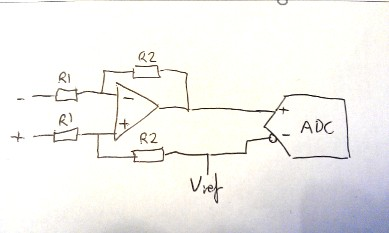# ADC + OP-AMP only generate positive numbers.

Hi.

I´m using:

Arduino + ADC (ADS1211P) + coil and magnet. Everything works great, when i move a magnet near the coil, i get positive and negative numbers. Measuring the voltage using coil+magnet, i get a range from -0.1v to 0.1v (or 0.01v?).

Since the signal is so small, i wish to amplify it using a OP-AMP.

So i added a non-inverting op-amp (5V single supply), measuring the output of amp is around 0v-5v. When i connect to the ADC, the output of the amp goes to one input, the second input from the ADC goes to REF 2.5V from the ADC.

The problem is: ADC only give positive numbers when moving the magnet near the coil.

When using Self calibrated mode in the ADC, the values start at 0 and goes up to +32000 (16bits). When not using calibration on the ADC the values start at -24000 and goes up to 32000.

I cant really understand where the problem isStrange thing, i´m getting 1.6V in any input in the ADC?! I´m only using a breadboard, just wish to do some tests..not looking yet for accuracy in the ADC.

Thanks.

A single-supply op-amp cannot supply negative voltage. You will need dual supplies.

Thats my idea too, but i ordered 2 years ago a small PCB board with the same ADC and OP-AMP and i get positive/negative numbers. Circuit ordered:

I also tried to replicate the above circuit and still cant get negative values (this is relative, if i connect the output of the amp to the other input, i will get negative values, but no positive ones).

Why cant i even replicate the circuit and get the same results? Maybe its from working in a breadboard and power the ADC/amp from USB?

Hi.

I figured out i need some kind of bias.. so i´m using a voltage divider in one of the inputs, with no gain control in the op-amp its seems to solve my problem, but when i add a non inverting circuit, the op-amp output only noise (steady 4.6V)..

the circuit i´m using:

-Orange wires -> input.
-IC is the A/D. One input of the A/D goes to Output from the OP-Amp, the other input goes to GND.

Fritzing file:

Anyone cant point where the issue is? I´ve all the stuff ready, just missing this op-amp issue..

Thanks

Since that ADC has differential inputs I would suggest using a differential amplifier circuit with
an op-amp, then feeding a 2.5V reference both to the ADC -ve input and as the reference input
to the differential amplifier.MarkT:
Since that ADC has differential inputs I would suggest using a differential amplifier circuit with
an op-amp, then feeding a 2.5V reference both to the ADC -ve input and as the reference input
to the differential amplifier.Thanks!! That circuit works perfectly, it solved my issueJust a last question.. using that circuit with the ADC set at 16bits, i´m only getting up +-16500, instead +-32000. Setting the internal ADC gain to 2x, i can get the correct up to +-32000, but why with internal ADC gain set to 1x, it wont go up to +-32000?